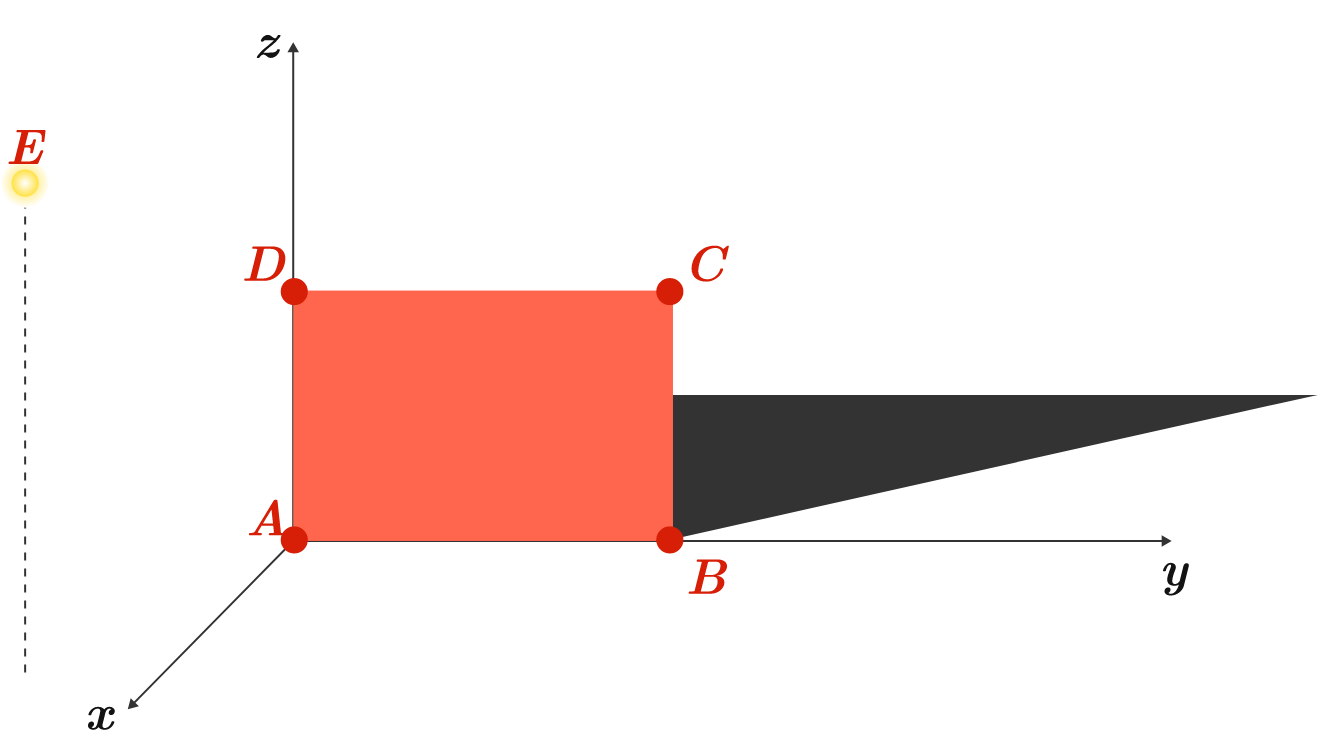# A light and a shadow

Geometry Level 2

A rectangular billboard $ABCD$ is illuminated by a lantern $E$ and casts a shadow in the $xy$-plane. The positions $(x,y,z)$ of the lantern and the billboard's vertices are $A = (0,0,0), \quad B = (0,3,0), \quad C = (0,3,2), \quad D = (0, 0 ,2), \quad E = (3, -1, 4).$ What is the area of the shadow?Assumptions: The light source is a point and the billboard has zero thickness. The ground is the $xy$-plane.

×

Problem Loading...

Note Loading...

Set Loading...